HomeenDiprotic Acid Definition

# Diprotic Acid Definition

A diprotic acid is a Brønsted and Lowry acid possessing two ionizable hydrogen atoms . That is, it is an acid capable of donating two protons when dissolved in water or when reacting with two molecules of a base; hence its name. In fact, di- is a prefix that means 2, while protic refers to the proton, in other words, the cation that forms the hydrogen atom by losing its only electron.

Diprotic acids can be both strong and weak, although the vast majority are weak acids. Even in the few cases where they are strong, such as sulfuric acid, only the first dissociation is strong, while the second dissociation is weak.

In view of the above, the vast majority of diprotic acids have two ionization constants or acid dissociation constants, K a1 and K a2 .

## General formula of diprotic acids

There are many examples of diprotic acids with widely varying chemical formulas. However, in the context of calculating pH or other calculations related to acid-base balance, they are usually represented by the generic formula H 2 A , where A represents the anion that forms when the acid loses both protons. The anion A can be as simple as a sulfide ion and as complex as an organic ion with tens of atoms.

## Acid-base balance of diprotic acids

As mentioned above, most diprotic acids are weak and only rarely have both a strong and a weak dissociation. This highlights the fact that having two ionizable protons does not mean that diprotic acids donate them simultaneously. In fact, dissociation is a sequential proton loss process that involves, in the case of diprotic acids, two chemical equilibria and therefore two acid dissociation constants K a1 and K a2 . The corresponding reversible chemical reactions are presented below:

### 1 st dissociation of a diprotic acid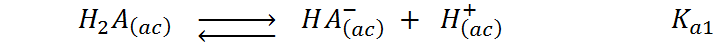### 2nd dissociation of a diprotic acid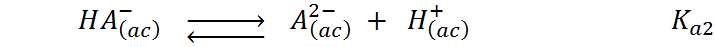## Calculation of pH of solutions of diprotic acids

The calculation of the pH and of the concentration of all the species present in a solution of a diprotic acid, given its initial or analytical concentration, implies simultaneously solving the ionic equilibria shown above. These types of problems are considerably complex, since these two equilibria are connected both through the concentration of protons (H + ) in the solution, and by the concentration of the conjugate base of the original acid (HA – ).

As can be seen in the equations of both equilibrium constants, there are a total of 4 unknowns to be found and they are [H 2 A], [HA – ], [A 2- ] and [H + ]. Since we only have two equations (the two equilibrium constants), two additional independent equations are needed to be able to solve this type of problem. Analytical chemists call these equations mass balance and balance of electric charges, which for this case are given by:

### Mass balance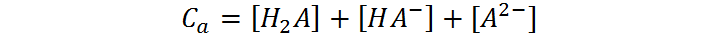This equation expresses that the total concentration of a diprotic acid, also called the analytical concentration (C a ), is distributed among all the ionized and non-ionized species of the acid, namely, H 2 A, HA – and A 2- .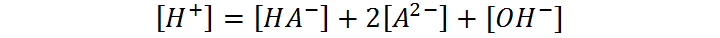This equation, for its part, expresses the condition of neutrality of the solution. Since the solution must be neutral, the sum of all the positive charges coming from all the cations present in the solution (in this case only the protons) must be balanced by the negative charges from all the anions.

Combining the equations for K a1 , K a2 , the dissociation constant of water (K ​​w ), the mass balance, and the charge balance, provides an equation that allows us to find the concentration of protons and, from there, that of all other species in equilibrium. This is a laborious and complicated process that is beyond the scope of this article; however, it can be solved approximately by neglecting the second dissociation and taking into account only the first.

## Examples of Diprotic Acids

Below are some examples of common diprotic acids. In the cases of organic acids, it must be taken into account that only the hydrogens of the carboxyl groups are ionizable, so those that are directly attached to carbon atoms are not taken into account as protons.

### Sulfuric acid (H 2 SO 4 )### Carbonic acid (H 2 CO 3 )### Terephthalic acid (HOOC-C 6 H 4 -COOH)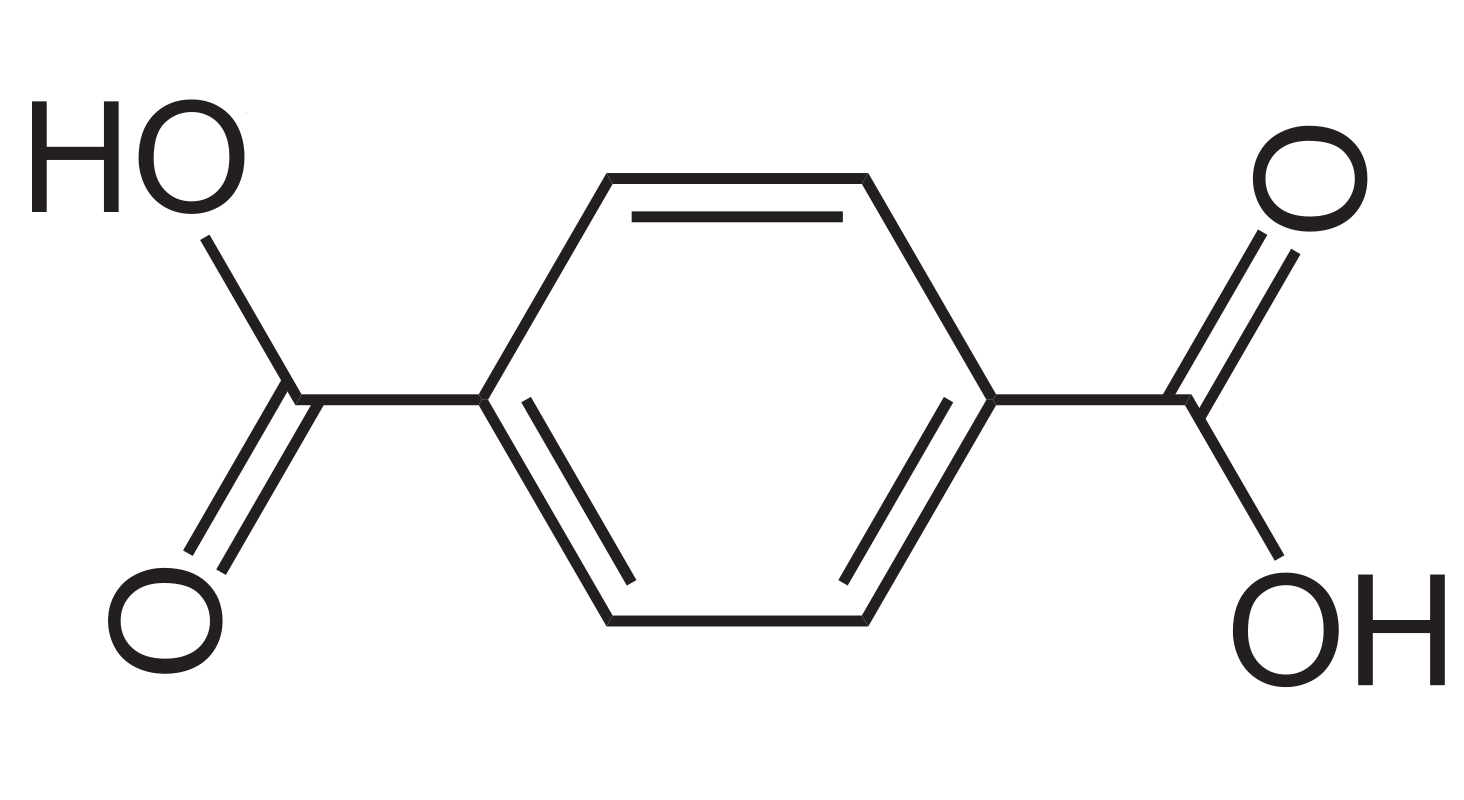### Other diprotic acids

• Chromic acid ( H 2 CrO 4 )
• Oxalic acid ( H 2 C 2 O 4 )
• Butenedioic acid ( HOOC -CH=CH- COOH )
• Hydrogen sulfide ( H 2 S)
• Hydrogen telluric acid ( H 2 Te)
• Selenhydric acid ( H2Se )

## References

Diprotic acid : definition , formula and examples . (2020, October 29). studying. https://estudyando.com/acido-diprotico-definicion-formula-y-ejemplos/

DIPROTIC AND POLYPROTIC ACIDS – Basic concepts . (nd). Dawn-temuco. https://www.amanecer-temuco.cl/wp-content/uploads/2020/05/4-Acidos-diproticos-y-poliproticos-Conceptos-basicos.pdf

Campillo, N. (2011). EQUILIBRIUM AND ACID-BASE VOLUMETRIES . University of Murcia. https://www.um.es/documents/4874468/11830096/tema-5.pdf/e9fc6f0c-2d0d-4bca-8b07-6e1f03ff7c30

Chang, R. (2021). Chemistry ( 11th ed.). MCGRAW HILL EDUCATION.

Examples of Diprotic Acids . (2015, October 8). Químicas.net. https://www.quimicas.net/2015/05/ejemplos-de-acidos-diproticos.html

Libretexts. (2020, October 30). 14.5: Polyprotic Acids . Spanish LibreTexts. https://espanol.libretexts.org/Quimica/Libro%3A_Quimica_General_(OpenSTAX)/14%3A_Equilibrio_de_acido-base/14.5%3A_Acidos_poliproticos